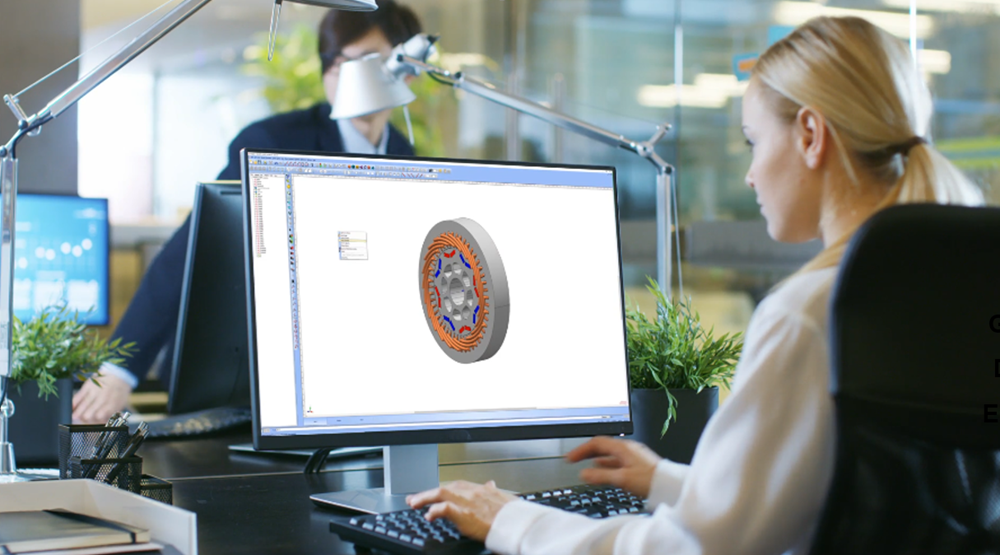# Improving Hysteresis and Eddy Losses in Electric MachinesDesigners of modern electric machines understand the importance of lowering the hysteresis and the eddy current losses. They are commonly referred to as core losses or iron losses. It is an important aspect of improving the overall efficiency of machines. In this blog I will discuss how some recent additions to Simcenter MAGNET 2020.1 and Simcenter Motorsolve 2020.1 will help designers improve the accuracy of these loss calculations.

## Complexity of Calculating Hysteresis and Eddy Losses in Machines

Iron losses are caused by material hysteretic behavior and eddy current losses. However, their prediction has been an area of tremendous research and development as it is one of the few avenues to explore when searching for that extra source of loss savings for modern electric machines. These calculations, however, are complex because no single model can represent the broad spectrum of physical conditions devices operate at.

In most commercial software, iron losses are calculated using empirical data that are fitted to a class of equations called the extended Bertotti (or Steinmetz-type) formulae. Below is a typical manufacturer supplied loss data and the formula it is fitted to in Simcenter MAGNET and Motorsolve.

Figure 1: Iron loss data fitted to an extended Bertotti formula for iron loss calculations.

The accuracy of the Bertotti approach is limited because of a number of phenomenological reasons. Ideally, designers should use a fully hysteresis solver based calculation for predicting iron losses. However, this approach is time consuming, and not necessarily the best option to apply during early stages of design when relatively quick results are needed, especially for 3D simulation problems.

The difficulty of applying the extended Bertotti formula arises because the fitting algorithm’s accuracy is not uniform across low to high flux density and frequency ranges.

To address this problem, in Simcenter MAGNET 2020.1 and Simcenter Motorsolve 2020.1, three data fitting options have been added:

• User defined specification of the hysteresis and the eddy current loss calculation coefficients (from testing or external experiments)
• Relative error loss
• Absolute error loss

When applied together, the relative and absolute error based algorithms are highly accurate, up to ~1.8 T flux density and ~2000 Hz frequency levels. This range covers much of the operating conditions for most modern electric machines.

## Hysteresis and Eddy Losses in an IPM Machine

For example, consider a 8 pole 48 slot interior permanent magnet motor. Simulating this machine under a few operating conditions will help illustrate the benefit of the different fitting options. The losses are calculated using the extended Bertotti formula and are compared to Simcenter MAGNET’s hysteresis field solver.

Figure 2: Cross section of the motor whose loss calculations are being done

The percentage difference between the hysteresis solver based calculation and the relative error based data fitting and the absolute error based approach has been shown on Table 1. The example illustrates the difficulty of selecting any given fitting method and applying it across the board under all operating conditions.

Table 1: Summary of percentage error between hysteresis solver results and iron loss data fitting algorithms

The results illustrate the fact that when applying these equations numerical experimentations using different fitting methods should be carried out to best determine their range of applicability. It will ensure that the right method is used at a given operating condition.

## Hybrid Data Fitting Approach

The calculation of hysteresis and eddy current losses in electric machines accurately is a complex area of research combining physics on very small scale, experiments on macro scale and computational electromagnetics.

In this blog using some new features of the Simcenter MAGNET and Motorsolve 2020.1 software I have discussed the difficulty of calculating these losses while showing how to improve upon existing methodologies. The need to consider a case specific hybrid data fitting approach when calculating iron losses has been shown.

Topics